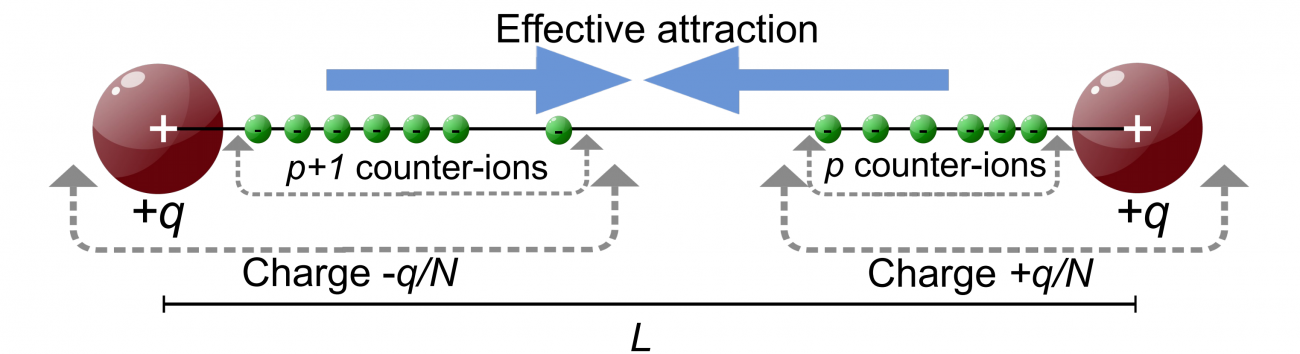2018-2021

# Coulomb systems in one and two dimensions: exact results

• Principal investigator(s):
• Funded by:
ECOS Nord (France)/Minciencias (Colombia)Physical models that have an exact solution play a particularly important role in science as they provide a solid theoretical framework for testing hypotheses and conjectures. In this project we intend to study a couple of models of fluid systems composed of electric charges (Coulomb systems) for which their thermodynamic properties can be determined analytically and accurately using the formalism of equilibrium statistical physics. A first model that will be studied is a one-dimensional system in which two fixed charges are shielded by mobile ions. We will determine in this model how is the effective interaction between the two charges and the ion configurations of lower free energy. A second model that we will study is the two-dimensional two-component plasma with charge asymmetry. This is a system made up of positive and negative particulars, but with different charges in a +1 / -2 ratio. This model has an exact solution. Using this we will determine how the correlation functions between charges behave at short distances and how this behavior changes when the electrostatic coupling parameter between charges varies.

`Universidad de los Andes | Vigilada MineducaciónReconocimiento como Universidad: Decreto 1297 del 30 de mayo de 1964.Reconocimiento personería jurídica: Resolución 28 del 23 de febrero de 1949 Minjusticia.`